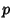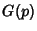## Rigid

A Framework is rigid Iff continuous motion of the points of the configuration maintaining the bar constraints comes from a family of motions of all Euclidean Space which are distance-preserving. A Graphis (generically)-rigid if, for almost all (i.e., an open dense set of) Configurations of, the Frameworkis rigid in.

One of the first results in rigidity theory was the Rigidity Theorem by Cauchy in 1813. Although rigidity problems were of immense interest to engineers, intensive mathematical study of these types of problems has occurred only relatively recently (Connelly 1993, Graver et al. 1993).

See also Bar (Edge), Flexible Polyhedron, Framework, Laman's Theorem, Liebmann's Theorem, Rigidity Theorem

References

Connelly, R. ``Rigidity.'' Ch. 1.7 in Handbook of Convex Geometry, Vol. A (Ed. P. M. Gruber and J. M. Wills). Amsterdam, Netherlands: North-Holland, pp. 223-271, 1993.

Crapo, H. and Whiteley, W. ``Statics of Frameworks and Motions of Panel Structures, A Projective Geometry Introduction.'' Structural Topology 6, 43-82, 1982.

Graver, J.; Servatius, B.; and Servatius, H. Combinatorial Rigidity. Providence, RI: Amer. Math. Soc., 1993.